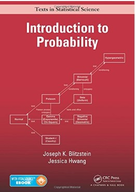Introduction To Probability - 1 Edition - Chapter 5 - Problem 19
Register Now

Join StudySoup

Get Full Access to Introduction To Probability - 1 Edition - Chapter 5 - Problem 199781466575578

# Let F be a CDF which is continuous and strictly increasing. Let be the mean of the

Introduction to Probability | 1st Edition

Problem 19

Let F be a CDF which is continuous and strictly increasing. Let be the mean of the distribution. The quantile function, F 1, has many applications in statistics and econometrics. Show that the area under the curve of the quantile function from 0 to 1 is . Hint: Use LOTUS and universality of the Uniform.

Accepted Solution
Step-by-Step Solution:
Step 1 of 3

Week 3 EDA for 2-Variable Data: - 2-variable data – when you have two measurements for each individual - An association (relationship) exists when values of a certain variable are more likely to occur when you have certain values of another variable - Explanatory variable is the x - Response variable is the y Explanatory Response Variable EDA Variable Categorical Quantitative Display: side-by-side boxplots (MORE DETAILS BELOW) Summary: descriptive statistics Categorical Categorical Display: contingency

###### Chapter 5, Problem 19 is Solved

Step 2 of 3

Step 3 of 3

Unlock Textbook Solution